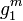# Encoding of external direct product in terms of encodings of direct factors

## Statement

This article describes how to construct an encoding of the external direct product$G_1 \times G_2$ given separate encodings of$G_1$ and$G_2$. Note that the nature of the encodings for$G_1$ and$G_2$ may be quite different.

For instance,$G_1$ may have a permutation encoding and$G_2$ may have a linear encoding. This does not matter -- we will still get an encoding of$G_1 \times G_2$ albeit it will be neither a permutation encoding nor a linear encoding.

## Encoding method

### New code-words

The idea is to encode$(g_1,g_2)$ by combining the code-words for$g_1$ and$g_2$. How the combination is done depends on our goals. Two possibilities:

• Easier possibility: Introduce a new symbol for a comma separator, then write the new code-word by writing the code-word for$g_1$, then the comma separator, then the code-word for$g_2$.
• Harder but in some cases more useful possibility: Alternate the code-word letters for$g_1$ and$g_2$.

### Algorithms

The idea behind each algorithm (identity element, multiplication, inversion, and membership test) for the direct product is as follows:

Shorthand for algorithm How it's executed
identity element We have algorithms for the identity elements$e_1$ in$G_1$ and$e_2$ in$G_2$. Just output the element$(e_1,e_2)$.
group multiplication Consider two input code-words$(g_1,g_2)$ and$(h_1,h_2)$ that need to be multiplied. First, parse to recover the code-words for$g_1,g_2,h_1,h_2$. Then, compute the products$g_1h_1$ and$g_2h_2$. Finally, output$(g_1h_1,g_2h_2)$.
inverse Parse the code-word for$(g_1,g_2)$ to get code-words for$g_1$ and$g_2$. Use the inversion algorithms within the groups$G_1$ and$G_2$ to find code-words for$g_1^{-1}$ and$g_2^{-1}$. Finally, output$(g_1^{-1},g_2^{-1})$.
membership test Parse the code-word for$(g_1,g_2)$ to obtain the code-words for$g_1$ and$g_2$. Then, check whether$g_1$ is a valid code-word for$G_1$ and also whether$g_2$ is a valid code-word for$G_2$.

Shorthand for algorithm Description of algorithm for$G_1 \times G_2$ in terms of algorithms for$G_1$ and$G_2$ separately
list all group elements (also called an enumeration algorithm) We use$G_1$ for the outer traversal loop and$G_2$ for the inner traversal loop. For each element$g_1 \in G_1$, list all pairs$(g_1,g_2)$ for all$g_2 \in G_2$.
order of the whole group multiply the known orders of$G_1$ and$G_2$. See order of direct product is product of orders
uniform random sampling from the whole group we can use separate uniform random samplers for$G_1$ and$G_2$ and then output the element we obtain by combining the answers: if the random element of$G_1$ is$g_1$ and the random element of$G_2$ is$g_2$, the random element of$G_1 \times G_2$ is$(g_1,g_2)$.
algorithm for the power computation problem Carry out the power computations separately in each coordinate. Explicitly, to find$(g_1,g_2)^m$, compute$g_1^m$ and$g_2^m$ separately, then combine.
algorithm for the element order-finding problem: computing the order of an element Compute the orders of the coordinates separately, then compute the least common multiple of the orders (if the orders come equipped with prime factorizations, this is easy; otherwise, use the Euclidean algorithm to compute the gcd and then compute the lcm from that).

A multi-encoding of a group is like an encoding, except that there could be multiple code-words for the same element, and we have a separate equality test to determine whether two code-words represent the same element. We can use the procedure described above to obtain a multi-encoding for$G_1 \times G_2$ in terms of multi-encodings of$G_1$ and$G_2$. The main difference is that we will need to add in an equality test that would first parse to obtain the coordinates, then check equality coordinate-wise.